• 1、采样频率和采样周期的...【Matlabfft的正确简单理解】 http://blog.sina.cn/dpool/blog/s/blog_166c28bd30102x7dg.html 【Matlab fftshift 详解】 https://blog.csdn.net/myathappy/article/details/51344618
1、采样频率和采样周期的设定： 采样频率的设定应满足奈奎斯特准则，当采样频率设定之后求倒数即为采样周期。 如： Fs = 1000; % Sampling frequency 采样频率 T = 1/Fs; % Sampling period 采样周期
2、采样点数的确定： – 若想要规定采样点数，则采用如下方式： L = 1000; % Length of signal 对信号的采样点数 t = (0:L-1)*T; % Time vector 采样的时间点序列 –若想要规定时间范围则采用如下方式： t = -5:T:5; L = length(t);
3、频率的分辨率： 时域的采样频率决定了频域的最大值（fmax=fs)，所以在已知信号的时域采样率后，可得频率域的分辨率ff，即ff=fs/N. 其中N为做FFT的点数，可以根据分辨率的需要来自行设定，在没有输入N的情况下系统默认为信号时域的采样点数L！但是注意设定的N值要产生的最小分辨率要小于被分析信号频率间的最小差值，要不然会失真！ 如： –不输入N时，fft(X)中N默认为为采样点数L。 –输入N时，fft(X,N)即对X做N（可自由设定）点fft。 N值虽可以自由设定，但N值越小，频率分辨率越大，当频率分辨率大于信号最小频率分量时，得到的频谱会失真。
4、频率轴的处理 频率轴的归一化： 经过N点FFT得到的频谱被分成N份范围为[0 fs],不是真实的频域。要想获得真正的频率轴，需要对频率轴进行归一化处理： f = Fs*(0:(N-1))/N; 频率轴归一化之后做平移（利用fftshift()）使其关于0对称： 做完fft之后在做fftshift(),同时对频率轴进行归一化和平移处理 Y = fft(X); Y1 = fftshift(Y); f = Fs*(((-L)/2):(L/2-1))/L;
参考代码： clc; clear; close;
%% 注释 % MATLAB_help注释 + 个人理解注释 Fs = 1000; % Sampling frequency 采样频率 T = 1/Fs; % Sampling period 采样周期 % 根据需要设定L和t序列；想要确定采样点数则用方式1；想要确定时间范围则用方式2 % L = 1000; % Length of signal 对信号的采样点数 % t = (0:L-1)*T; % Time vector 采样的时间点序列 t = -5:T:5; L = length(t);
%% 对信号进行采样 S = 0.7sin(2pi50t) + sin(2pi120t); X = S + 1randn(size(t));
%% 对采样信号做一系列FFT处理 fft(x)默认做L点的FFT，L为采样点数 figure(1); plot(1000*t(1:100),X(1:100)) title(‘Signal Corrupted with Zero-Mean Random Noise’) xlabel(‘t (milliseconds)’) ylabel(‘X(t)’)
% 对加噪声信号做FFT Y = fft(X); Y1 = fftshift(Y); figure(2) plot(abs(Y)) title(‘未做归一化处理的FFT结果’)
P2 = abs(Y/L); f = Fs*(0:(L-1))/L; figure(3); plot(f,P2) title(‘Single-Sided Amplitude Spectrum of X(t)’) xlabel(‘f (Hz)’) ylabel(’|P1(f)|’) legend(‘fft变换后，归一化且对频率进行正确化后的频谱图’);
P1 = P2(1:L/2+1); % P1(2:end-1) = 2P1(2:end-1); f = Fs(0:(L/2))/L; figure(4); plot(f,P1) title(‘Single-Sided Amplitude Spectrum of X(t)’) xlabel(‘f (Hz)’) ylabel(’|P1(f)|’) legend(‘fft变换后，归一化且对频率进行正确化后，取一半的 频谱图’);
f = Fs*(((-L)/2):(L/2-1))/L; figure(5); plot(f,abs(Y1)/L) title(‘Single-Sided Amplitude Spectrum of X(t)’) xlabel(‘f (Hz)’) ylabel(’|P1(f)|’) legend(‘fft变换后，归一化且对频率进行正确化后，并做了频谱搬移 频谱图’);
%% 对采样信号做一系列N点的FFT处理 fft(x,N) N = 128; y1 = fft(X,N); y1 = abs(y1)/N; f = Fs*(0:(N-1))/N; plot(f,y1) title(‘Single-Sided Amplitude Spectrum of X(t)’) xlabel(‘f (Hz)’) ylabel(’|P1(f)|’)
程序对应的仿真结果：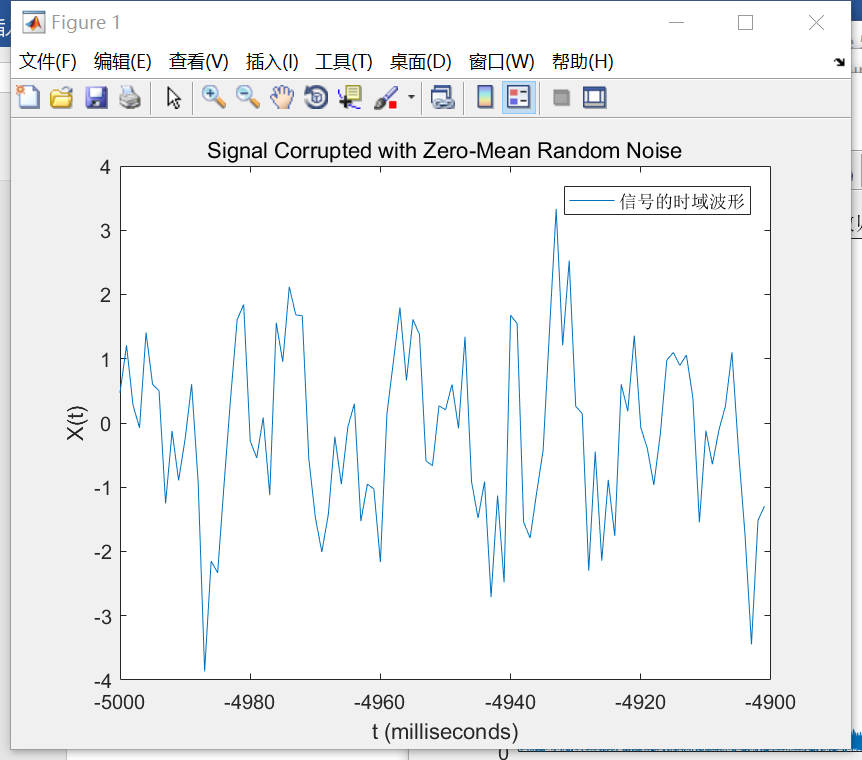为了便于显示，仅取时域波形的一段进行显示。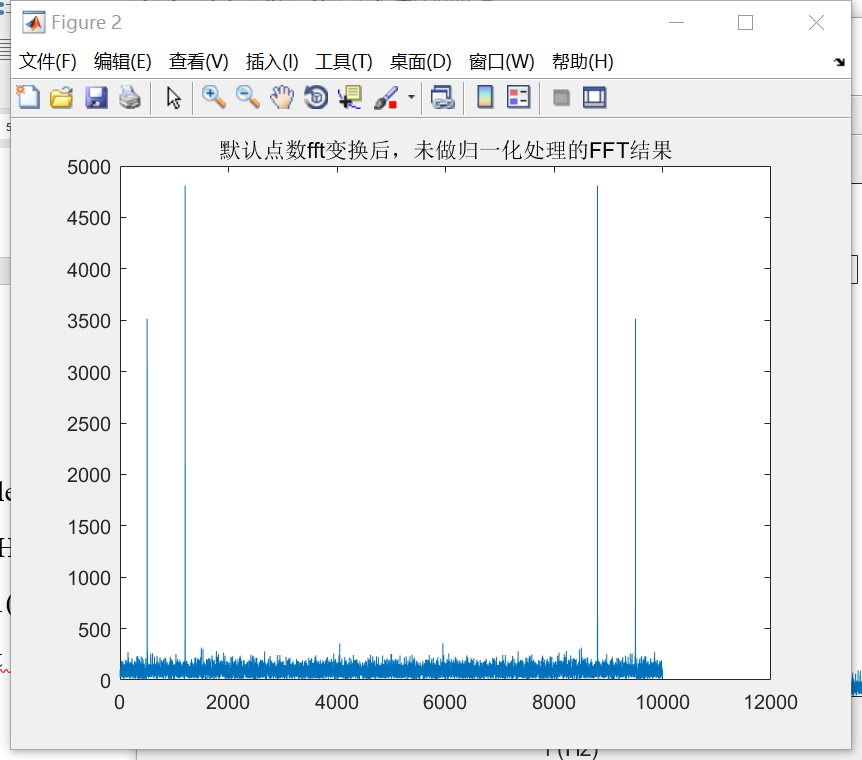从图中可以看出，未做归一化处理之前，纵轴值很大，横轴对应着fft的点数。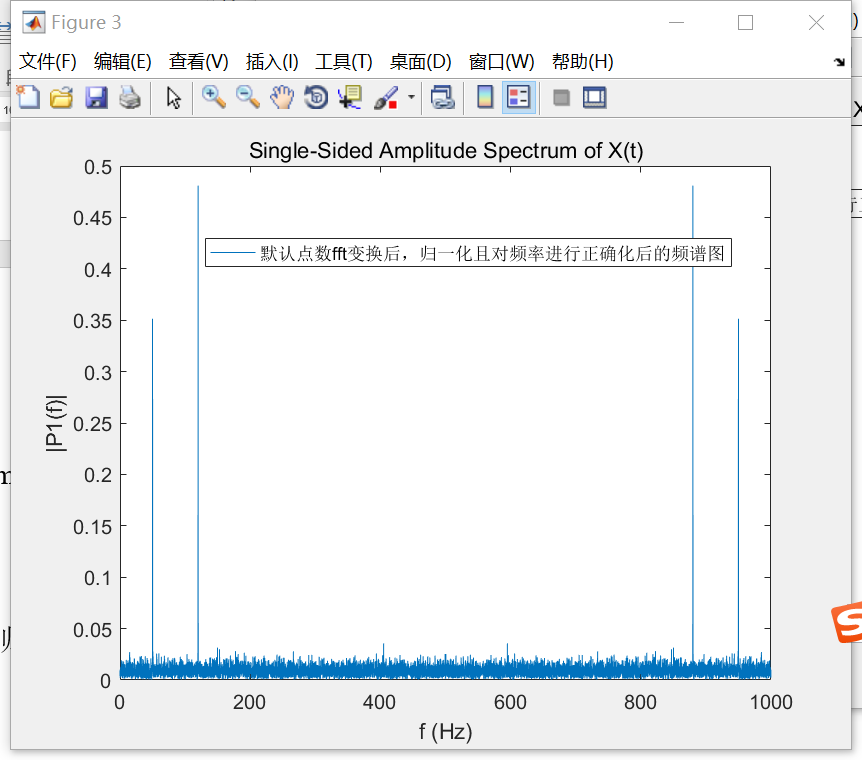从图中可以看出，归一化处理之后，频谱图的范围为[0 fs]，但实际的频谱范围应该为[-fs/2  fs/2],所以还需要做一下平移，如下图所示。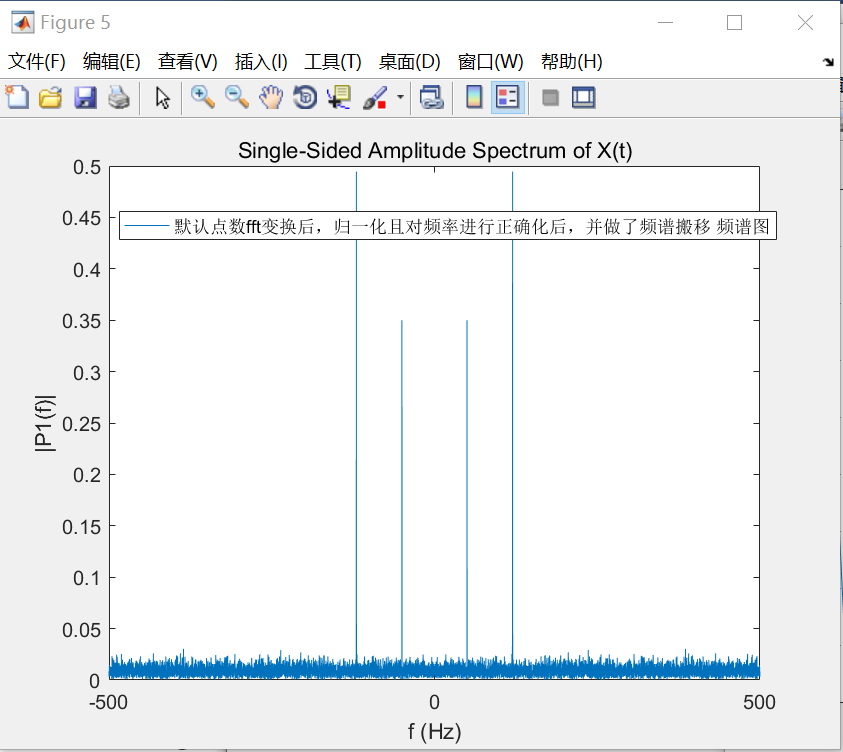当进行N点fft时，N过小时会使频谱图失真，如下图所示。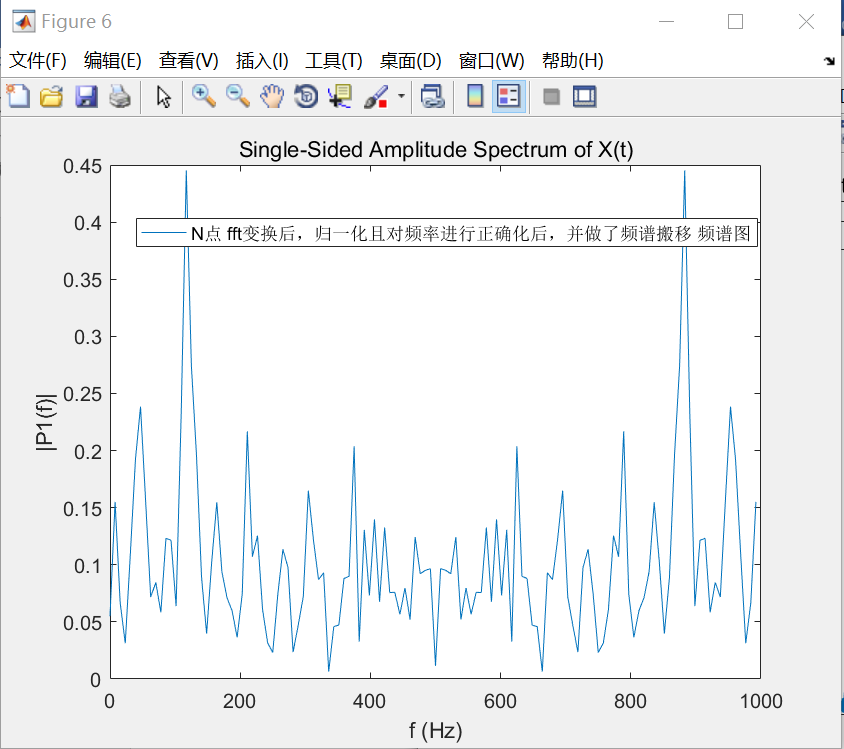参考网址： 【Matlab中fft的正确简单理解】http://blog.sina.cn/dpool/blog/s/blog_166c28bd30102x7dg.html 【Matlab fftshift 详解】https://blog.csdn.net/myathappy/article/details/51344618
展开全文• 基于matlabFFT算法实现实序列线性卷积方法二-ditfft.m 基本思想上一贴已经说过只要按照程序运行即可 分块执行，特别要说明的是该倒序算法比较经典 方法很是独特，注意体会 附件中 第一个为倒序算法，第二个是...
• ## MATLAB实现FFT算法

千次阅读 2020-04-05 08:32:09
MATLAB实现FFT算法项目简述项目代码项目仿真结果总结 项目简述 傅里叶变换是信号处理中最重要的概念之一。其中，傅里叶变换是针对连续信号变换到频域的数学方法。但是在数字化的时代，我们都会将连续信号进行抽样，...


MATLAB实现FFT算法
项目简述项目代码项目仿真结果总结

项目简述
傅里叶变换是信号处理中最重要的概念之一。其中，傅里叶变换是针对连续信号变换到频域的数学方法。但是在数字化的时代，我们都会将连续信号进行抽样，进而得到相应的离散信号。抽样又可以根据抽样脉冲的不同分为理想抽象、脉冲抽样等。连续域里面的傅里叶变换经抽样之后变成了离散时间傅里叶变换（DTFT），我们对DTFT进行频率抽样变成离散傅里叶变换（DFT）。于是我们便可以从DFT中观察离散信号的频率成分，但是DFT需要的计算量特别大，不适合硬件的实现，于是研究人员针对DFT的特点提出了快速傅里叶变换（FFT）。这里需要注意，FFT只是DFT的快速实现，并不是一个新的变换。相信同学们从上面的概念中可以明白信号处理的发展过程。
我们本次实验是生成两个正弦波，先进行混频，然后进行FFT观察频率分量。
项目代码
本次实验所用到的代码如下：
clc;
clear all;
FS = 100000000;
FT1 = 5000000;
FT2 = 8000000;
n = 0:1:1023;
f1 = sin(2*pi*FT1*n/FS);
f2 = sin(2*pi*FT2*n/FS);
f = f1.*f2;
F = fftshift(abs(fft(f)));
plot(-512:1:511,F)

项目仿真结果
我们运行上面的MATLAB文件，可以得到下面的现象：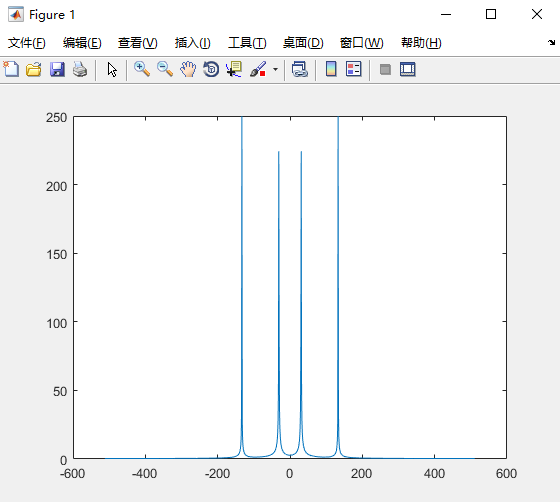经过上面的仿真结果，我们观察0到511个点有两个脉冲分别代表了混频之后的两个频率，从而证明了我们实验的正确性。
总结
创作不易，认为文章有帮助的同学们可以关注、点赞、转发支持。（txt文件、图片文件在群中）对文章有什么看法或者需要更近一步交流的同学，可以加入下面的群：展开全文fpga
• 利用空闲时间熟悉了一下MATLAB中的FFT的使用。 相信绝大多数初学者都和我一样，对FFT输出的结果具体是什么很困惑，搞不明白怎么处理，尤其是幅度的处理，这里我针对FFT输出的数据主要涵盖的几个问题实验分析了一下...
利用空闲时间熟悉了一下MATLAB中的FFT的使用。
相信绝大多数初学者都和我一样，对FFT输出的结果具体是什么很困惑，搞不明白怎么处理，尤其是幅度的处理，这里我针对FFT输出的数据主要涵盖的几个问题实验分析了一下。
1、关于单边带双边带的问题:
matlab中fft函数的输出结果是一个双边带的数据，先贴出实验中的一个图看一下：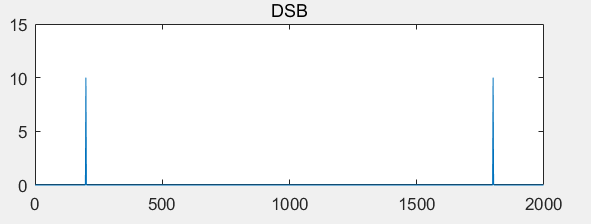然而实际中我们只需要用到其中的一半，因此我们在最后的分析中只需要取出1~N/2+1这前N/2+1个点来分析就好了。这个双边带信号是根据N/2+1对称的。
2、幅度修正
幅度修正问题，通过如下公式就能理解（图来源于网络，侵删）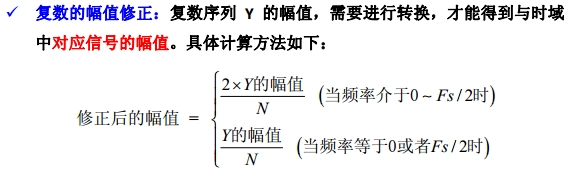即，在频率点为基波和Fs/2时，对应的时域幅度等于FFT输出的幅度的1/N，在这两者之间的时候，幅度还要乘2.
因此对输入的序列做FFT之后，只需要做两步操作就可以得到我们需要的结果：
a、双边带转单边带；
b、幅度修正（基波和其他频率点差2倍关系）
代码：
close all;clear;clc;

%%
%parameter interface
SampleLen = 992;%Sampling number N
SampleFre = 2000;%Sampleing Frequence
SignalFre1 = 200;%signal frequence 1
SignalFre2 = 100;%signal frequence 2
t = (0:1:SampleLen-1)/SampleFre;
Signal_Input = 10*sin(2*pi*SignalFre1*t) + 0*sin(2*pi*SignalFre2*t);%add above 2 signal
Signal_Noise = 0 * rand(size(t));
Signal_Total = Signal_Input + Signal_Noise;%add noise
%Signal_Total = round(Signal_Total);
%%
FFT_Result = fft(Signal_Total);
FFT_Amplitude0 = abs(FFT_Result)/SampleLen;
FFT_Amplitude1 = FFT_Amplitude0(1:SampleLen/2+1);
FFT_Amplitude1(2:SampleLen/2+1) = 2*FFT_Amplitude1(2:SampleLen/2+1);%SSB
FFT_Amplitude0(2:end-1) = 2*FFT_Amplitude0(2:end-1);%DSB

%%
f_SSB = (0:SampleLen/2)*SampleFre/SampleLen;
f_DSB = (0:SampleLen-1)*SampleFre/SampleLen;
figure
subplot(3,1,1)
plot(t,Signal_Total);
title('Singnal_input');
subplot(3,1,2)
plot(f_DSB,FFT_Amplitude0);
title('DSB');
subplot(3,1,3)
plot(f_SSB,FFT_Amplitude1);
title('SSB');
实验结果：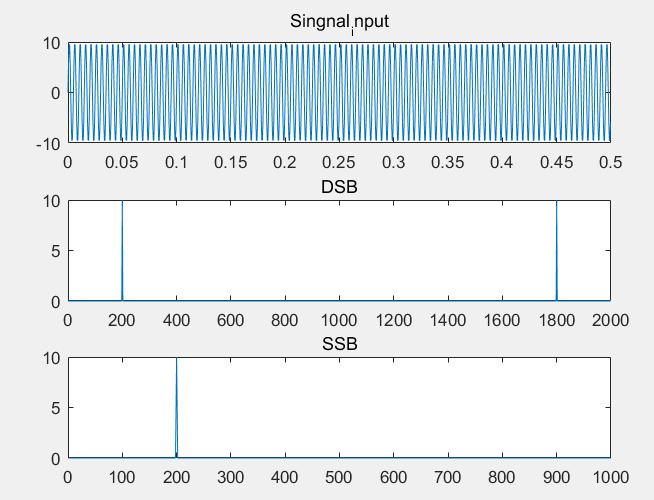最后关于频谱泄露的问题，我这里就不具体分析具体的可以参考其他博主https://blog.csdn.net/u014122266/article/details/43242905
根据这个博主的总结中关键的一句话是：非整周期截断是发生频谱泄露的充分且必要条件！
我个人也觉得，如果输入序列是非整周期的时候，是会发生频谱泄露的，但是这个说法被另一个博主在博文中否认：
https://blog.csdn.net/ciscomonkey/article/details/84679424，该博主通过实验，选取非整周期的序列验证发现并没有发生频谱泄露，据此我自己也做了一个非整数周期序列的实验，发现当输入的序列点数等于FFT的点数的情况下，只要输入的序列是非整数周期，还是会存在频谱泄露的情况，至于这个博主的内容我也就没深入理解了，有时间再看看我是不是有遗漏的细节。
下面贴出结果：
利用上面的程序，保持其他参数不变的情况下，当SampleLen=1000时：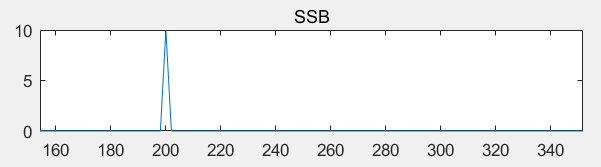当修改SampleLen = 992时：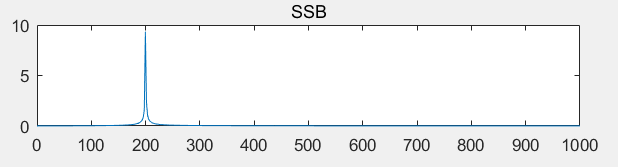上面两个图可以看出，的确是发生了频谱泄露。进一步修改SampleLen=990，为周期整数倍：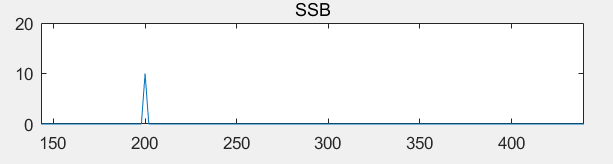频谱泄露的现象就没了。
因此根据我的实验现象看，本人的结论如第一个参考博主：非整周期截断是发生频谱泄露的充分且必要条件！

展开全文• ## Matlab 计算 FFT 的方法及幅值问题

万次阅读 多人点赞 2020-04-12 16:20:33
欢迎转载，但请一定要给出原文链接，标注出处，支持原创！...3.2 直流分量、噪声分量对 FFT 图像的影响3.3 总结 1、Matlab FFT 函数介绍   FFT (Fast Fourier Transform) 中文为快速傅里叶变换，作用是将离...
欢迎转载，但请一定要给出原文链接，标注出处，支持原创！ 谢谢~ https://blog.csdn.net/qq_29225913/article/details/105467006

目录
1、Matlab FFT 函数介绍2、Matlab FFT 程序3、FFT 程序分析3.1 fs 、N 对 FFT 图像幅值的影响。3.2 直流分量、噪声分量对 FFT 图像的影响3.3 总结

1、Matlab FFT 函数介绍
FFT (Fast Fourier Transform) 中文为快速傅里叶变换，作用是将离散的时域信号变换到频域，在一般情况下，时域信号都比较难看出特征，但是转换成频域后，就比较容易看出来，因此，很多信号分析都会采用FFT变换，然后对信号进行频谱分析。   Matlab 中，FFT 函数的语法如下：   Y = fft(X)   Y = fft(X,n)   Y = fft(X,n,dim) 推荐直接在命令行使用 help fft看到更加详细的描述信息与例程，这里主要记录一下用法以及注意事项。   N个采样点，经过FFT之后，就可以得到N个点的FFT结果。为了方便进行FFT运算，通常N取2的整数次方，但是这里直接取读取出来的样本数作为N。
FFT 计算公式如下：Xk 长度与 N 相同。根据奈科斯特定律，只有 f=fs/2 范围内的信号才是被采样到的有效信号，因此得到的频谱肯定是关于 N/2 对称的（就是只看前一半的波形就好）。第k点的实际频率的计算为 f(k) = k * (fs / n) — — (横轴的频率范围为 ：f = n * fs / N;)X 为直流分量 ，幅值 = 模值(X) / NX[k] 为个点的频率分量（除X外），幅值 = 模值(X[k]) / (N / 2)
2、Matlab FFT 程序
先摆上代码，运行一下看效果。 %%%%%%%%%%%%%%%%%%%%%%%%%%%%%%%%%%%%%%%%%%%%%%%
%   功能：FFT 运用方法（例程）                  %
%   作者：Mr-Ma Technology(马健维)             %
%   时间：2020.04.12                           %
%   转载请注明出处                             %
%   https://blog.csdn.net/qq_29225913/article/details/105467006
%%%%%%%%%%%%%%%%%%%%%%%%%%%%%%%%%%%%%%%%%%%%%%%

%% 描述
% 使用 Matlab 的 FFT，有一个注意事项，采样数量是采样频率的整数倍，且最好是 2 的 n 次幂。
% 否则会导致采样分辨率匹配不上，表现为在对应的频点处没有采样到，出现偏移，因此会导致
% 描绘图像时，发现所需要的频点处显示出来的幅度值小于预期的值。
% 可以通过修改参数定义的值得定义来直观的看FFT的效果

%% 参数定义
A1 = 2;             % 信号1 幅值（A）
f1 = 11;            % 信号1 频率（f）
A2 = 0.5;           % 信号2 幅值（A)
f2 = 29;            % 信号2 频率（f)
A3 = 1;             % 信号3 幅值（A)
f3 = 51;            % 信号3 频率（f)
Dc = 0;             % 直流分量
Noise = 0;          % 噪声大小
fs = 128;           % 采样频率 （fs）
N = 1024;           % 采样点数（样本数量）

%% 运算（生成时域曲线、FFT计算）
n = 0:N-1;          % 等差生成序列，
t = n / fs;         % 时间序列，用于下式
RA = rand(1,N);     % 随机噪声（0~1）
RA = RA - mean(RA); % 减去噪声均值（-0.5~0.5）,去除噪声中的直流分量。
x = Dc + A1 * sin(2*pi*f1*t) + A2 * sin(2*pi*f2*t) + A3 * sin(2*pi*f3*t)+ Noise * RA ; % 两个正弦信号、直流分量、噪声 相叠加

y = abs(fft(x,N));  % 对上式进行 N 点 FFT 计算 ，并取模值
A = y * 2 / N;      % 模值转换为幅值
A(1) = A(1) / 2;    % 根据公式 ，X 不用乘以 2
f = n * fs / N;     % 转换为频率区间

%% 绘图
subplot(2,1,1);
plot(t,x);
xlabel('时间/s');ylabel('振幅');
title('时域曲线')
subplot(2,1,2);
plot(f(1:N/2),A(1:N/2)); % 描绘图像
xlabel('频率/Hz');ylabel('振幅');
title(['幅频特性曲线 :Fs = ',num2str(fs),', N = ',num2str(N)])
  运行图片：3、FFT 程序分析
通过运行上面的代码，可以得到与上图一致的运行效果，该程序中，原函数是由 直流分量、信号1、信号2、信号3、噪声分量 叠加而成的（上图中暂时将直流分量与噪声分量设置为0），在实际工程使用中，将例程中生成的原函数替换成需要进行分析的序列就可以了。   上面的图片中，每一个信号的幅值就刚好等于所设置的振幅，此处有一个地方需要注意的就是，一般情况下，采样点 N 都不固定，都是随输入的因素影响，例如对一首采样率为 44.1KHz 的歌来分析，读取出来的数据长度 N 就不一定是 2 的n次方了，如果直接送入进行 FFT 计算，到 N 点结算数据，在FFT内由于使用蝶形运算，所以是需要 N 是 2 的 n 次方，因此会导致在 N 个采样点后方补零， 可能在某些频点出看到的赋值，会比预想值要小，这就是能量泄露。如果只需要考虑各频谱值得比例（即只看频率分布情况），不考虑实际 FFT 后的幅值的话，可以直接传入N进去，如果需要进行频谱分析，就要要考虑实际的幅值对分析结果的影响，此处就可以选择加窗，还有一种情况就是 N 足够大，并且是均匀分布，就可以考虑截取 N 的值，使得满足 N 等于 2 的 n 次方。
3.1 fs 、N 对 FFT 图像幅值的影响。
上述程序中，fs = 128；N = 4096；（N 是 fs 的整数倍，且 N 是 2 的 n 次方），下面来分析下不同的 fs 与 N 对实际 FFT 结果的影响。
1、 fs = 100，N = 100（这个情况下，信号 3 不满足 fs ≥ 2f 的情况，因此信号 3 不会显示出来）2、 增大 N ，fs = 100，N = 150，由于补零了，所以存在能量泄漏，计算出来的幅值会变小。3、 增大Fs，fs = 200，N=150，此时由于 N 与 fs 仍然是非整数倍关系，且样本数量不足够大，导致分辨率不足，使得不能够将所有的频率都能够对应上，造成频率泄漏，导致幅值变小。4、 继续加大 N ，直接扩大10倍，fs = 200，N = 1500，可以观察到随着样本数量增加的时候，幅频特性曲线会变窄，与实际的频率越接近，即精度越高。但是由于 fs 与 N 仍然非整数倍关系，因此仍然会有上一点存在的问题。5、 这一步，减少 N ，但是设置为 fs 的整数倍（往上滑，与第1点对比），fs = 100， N = 200，可以看出，与第 1 点对比的时候，幅值依然是实际值，但是精度更高了（由于N 的数多了一倍）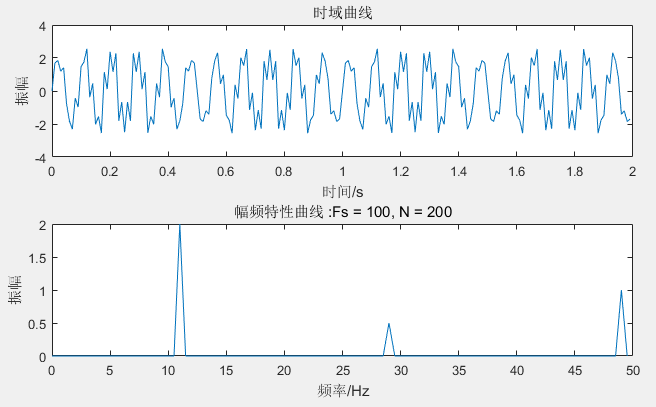6、 （对照组）扩大 N 为 fs 的 2 倍（与第 3 、5 点对比）fs = 200，N= 400，因为同样 N 是 fs 的整数倍，幅值为实际值。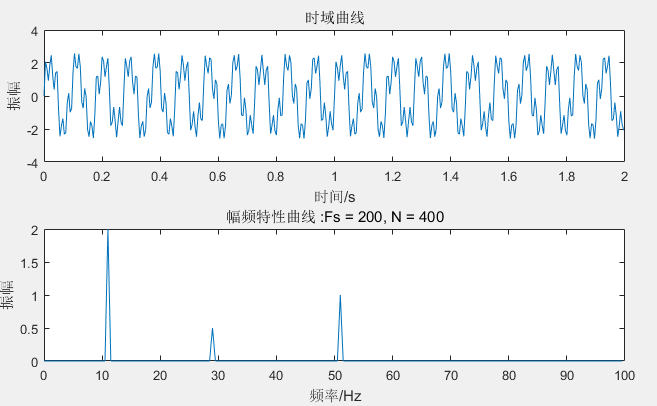7、 最后，设置 fs、N 均为 2 的 n 次方，这里的 fs 根据最高信号频率来选择即可，比如信号 3 是频率最高的信号（51Hz），因此 fs 选择128，N尽量的大，因此 N 选择 4096，此时时域信号中的频率信息，都能够很好的表示出来。3.2 直流分量、噪声分量对 FFT 图像的影响
在这一节考虑将直流分量，与噪声信号加入，通过改变以下两项来加入。其他值使用默认的参数(fs = 128，N = 1024)设置直流分量为1，可以看到 FFT 图像 x = 0 处有一个直流分量。(实际使用中，直流分量对信号的分析用处不大，所以一般都会将此值设为0，或者将 用 x = x - mean(x)的方法，将直流分量过滤掉)按照直流分量的计算公式，需要在程序里对该值进行修改（幅值 = 模值 / N ； 当 x = 0)修改噪声分量的值 为10，（即叠加一个 幅度为 ±5 的噪声信号），使用噪声前，需要对噪声进行消除直流处理。可以看出来，在时域的图像上，已经看不清信号的内容了，但是在频域上，仍然能够分辨的出有3个主要信号，并且在采样范围内，均匀分布着噪声信号。后面可以通过设计带通滤波器，将3个有用信号的频段提取出来，其他频段的信号进行过滤，在利用 IFFT 还原出降噪后的波形。3.3 总结
在能够自己选定 fs 的情况下，尽量选择 fs 为 2 的 n 次方，且 N 取 fs 的倍数，这样可以在幅频特性曲线上，得到与实际相符的幅度值。fs 固定的情况下，（如音频文件，44.1K/48K/…）,不能修改fs，则选择 N 为 fs 的整数倍，通常这种情况下 N 的数量都会足够大，因此 N 向前截取（即N值 ≤ 实际采样点）默认信息是随机分布，最后的一节信号不会对整体的频谱产生较大的影响。（若要考虑全部采样点，则可忽略对实际幅值的影响，实际幅值不一定需要）叠加噪声的分析，可以转换到频域上进行处理。
展开全文傅立叶分析 算法 经验分享
• ## Matlab实现FFT变换

万次阅读 2016-11-21 20:17:32
FFT信号流图：   程序实现是这样：   程序流程如下图：     首先进行位逆转，其实很简单，就是把二进制的位逆转过来： Matlab的位逆转程序： function a=bitreverse(N快速傅里叶变换
• 关于这个问题，在很早之前就分享过，也通过了解实现了算法，当时看的明白，想的明白，突然要用的时候，...matlab FFT 横坐标问题：前人关于FFT横坐标的详细阐述 我们知道Fourier分析是信号处理里很重要的技术，matl...
• FFT是离散傅立叶变换的快速算法，可以将一个信号变换到频域。... 虽然很多人都知道FFT是什么，可以用来做什么，怎么去做，但是却不知道FFT之后的结果是什意思、如何决定要使用多少点来做FFT。 现在就
• v1.0 可编辑可修改 按时间抽取的基 2FFT算法分析及 MATLAB实现 一 DIT-FFT 算法的基本原理 基 2FFT算法的基本思想是把原始的 N点序列依次分解成一系列短序列充分利用旋转因子 的周期性和对称性分别求出这些短序列...
• 以前一直对MATLABfft（）函数的使用一直存在疑惑，为什么要加一 些参数，并且如何确定这些参数，也查了许多资料，但很多都感觉只是 表面一说根本没有讲清其本质。但随着学习的推进，慢慢有所领悟，所 以打算把...
• matlabfft移植到python 1. 缘起 matlab源代码由一名大佬编写而成，其中的原理是蝶形运算。（我不太懂只能移植过来用了，以此加深对fft的理解）。 该文章链接：Peter_831 %----------------函数调用方法：Myfft...python
• ## matlabFFT 横纵坐标

千次阅读 2013-12-09 12:15:13
关于这个问题，在很早之前就分享过，也通过了解实现了算法，当时看的明白，想的明白，突然要用的时候，又...matlab FFT 横坐标问题：前人关于FFT横坐标的详细阐述 我们知道Fourier分析是信号处理里很重要的技术，matl
• matlabfft的用法及注意事项_调查/报告_......C 语言、MATLAB 实现 FFT 几种方法 总结前人经验,仅供参考 ///一...用matlab 编程: 构造一个信号函数 x 用 ? A * sin(2 * pi * f * t ? phi ) , FFT 变换后, 做出其...
• ## FFT在matlab中的使用方法

万次阅读 多人点赞 2019-10-04 16:29:30
FFTmatlab中的用法 一、FFT的物理意义 ​ FFT是离散傅立叶变换的快速算法，可以将一个信号变换到频域。有些信号在时域上是很难看出什么特征的，但是如果变换到频域之后，就很容易看出特征了。这就是很多信号分析...
• ## FFT在MATLAB画图步骤

千次阅读 2017-06-15 10:51:59
FFT总结步骤： 1.确定采样频率fs 一般是信号频率的2-5倍  2.确定采样点数N 一般是信号的周期T乘以fs  fs=4*fc; %采样频率，fc信号频率  Ts=1/fs; %一个周期采样时间  N=T/Ts; % 信号长度，T*fs  3...
• 作为一个资深的健忘症患者，需要把每次用都忘记的FFT问题进行整理。 FFT可将信号从时域转换到频域。 首先是一些简单常识： 采样周期：两次采样之间的时间间隔。 采样频率：1/采样周期。每秒采样的点数。（注意：...
• ## MATLAB的FFT变化总结

千次阅读 2015-03-15 19:56:51
1、FFT输出意义 FFT（x）变化不指定向频域变化时的采样点个数，如果时域数据点的个数为n,此时得到的是n个点的复数。 FFT（x,N）指定向频域变化时的采样点个数N，此时得到的是N个点的复数。 每个点横坐标对应一个...
• ## 用MATLAB进行FFT分析

万次阅读 2016-11-20 17:05:44
﻿﻿ 现在简单地介绍利用MATLAB进行快速傅里叶分析（FFT）。在工程中，常常需要在频域...以下归纳总结两种对已知数据进行傅里叶分析的方法：1熟悉电力电子MATLAB仿真的同学可以利用powrgui里面的FFT工具进行分析；2
• 自己整理的数字信号处理相关matlab程序，并对重要语句进行了解释、注解。设计卷积运算，滤波，滤波器的设计，以及极零图分析等有关数字信号处理的matlab文件。fftfilt
• IV、熟悉MatLab编程。 2.2实验原理 一个连续信号Xa(t)的频谱可以用它的傅里叶变换表示为： 如果对该信号进行理想采样，可以得到采样序列： 同样可以对该序列进行z变换，其中T为采样周期： 当z=e^jω的时候，我们...数字信号处理
• 总结：假设采样频率为Fs，采样点数为N，做FFT之后，某一点n（n从1开始）表示的频率为：Fn=(n-1)*Fs/N；该点的模值除以N/2就是对应该频率下的信号的幅度（对于直流信号是除以N）；该点的相位即是对应该频率下的信号...
• C程序编写的FFTmatlab中的fft函数比较 一、FFT的C程序编写 在上一次已经讲过如何用C语言编写一个FFT变换，不清楚的可以打开下面这个链接观看 https://blog.csdn.net/YAOHAIPI/article/details/102307425 经过...
• 利用matlabfft变换，可将其转换到频域中进行分析，代码如下： fs = 2*10; %根据采样定理，fs为你所关心的最大频率范围的两倍 L = length(0:1/fs:1);%对应图的采样点数 N = 2^nextpow2(L); %采样点数设置为2的整数...
• MATLAB教学视频，信号处理与系统分析类：本期视频时长约60分钟，首先回顾傅里叶变换的基本...通过具体的案例，深入讲解MATLAB FFT频谱的对称性和频率刻度的设置，总结归纳MATLAB FFT半谱图 & 全谱图的绘制方法和步骤。
• 毕设是图像处理方面的，在根据文献实现二维傅里叶变换时，因c++输出结果一直与MATLAB输出的不同，所以屡屡碰壁。 结果今天突然搞对了，很开心。 其实网上有实现代码，但因为没有明显以输出例子对比的，所以其实很多...opencv 计算机视觉
• 希望大伙喜欢！学数字图像处理的基础！matlab源程序
• FFT是离散傅立叶变换的快速算法，虽然很多人都知道FFT是什么，可以用来做什么，怎么去做，但是却不知道FFT之后的结果是什意思、如何决定要使用多少点来做FFT。现在说说FFT结果的具体物理意义。能量谱
• matlabFFT 函数的使用 学习记录 1、学习启示 N=1024; %采样点数为1024 Fs=1000; %采样频率为1000Hz t=[0:1/Fs:(N-1)/Fs]; %采样时刻 t的长为N s=2+3*cos(2*pi*200*t+60*pi/180)+4*cos(2*pi*300*t+120*pi/180); %......

# matlab的fft总结matlab 订阅